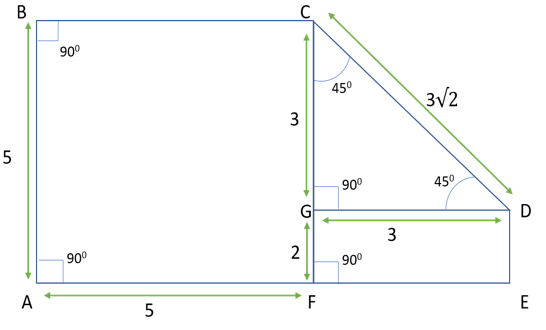GMAT Question of the Day - Daily to your Mailbox; hard ones only

 It is currently 23 Oct 2019, 22:01GMAT Club Daily Prep

Thank you for using the timer - this advanced tool can estimate your performance and suggest more practice questions. We have subscribed you to Daily Prep Questions via email.

Customized
for You

we will pick new questions that match your level based on your Timer History

Track

every week, we’ll send you an estimated GMAT score based on your performance

Practice
Pays

we will pick new questions that match your level based on your Timer History

Not interested in getting valuable practice questions and articles delivered to your email? No problem, unsubscribe here.In the figure above, the area of square ABCF is 25 and triangle CGD is

 new topic post reply Question banks Downloads My Bookmarks Reviews Important topics
Author Message
TAGS:

Hide Tags

Math ExpertV
Joined: 02 Sep 2009
Posts: 58472
In the figure above, the area of square ABCF is 25 and triangle CGD is  [#permalink]

Show Tags00:00

Difficulty:15% (low)

Question Stats:95% (01:25) correct5% (01:51) wrongbased on 60 sessions

HideShow timer StatisticsIn the figure above, the area of square ABCF is 25 and triangle CGD is an isosceles right triangle. What is the area of rectangle DEFG?

A. 15
B. 12
C. 9
D. $$6\sqrt{2}$$
E. 6

Attachment:2018-03-16_1012.png [ 8.33 KiB | Viewed 915 times ]

_________________
Math ExpertV
Joined: 02 Sep 2009
Posts: 58472
Re: In the figure above, the area of square ABCF is 25 and triangle CGD is  [#permalink]

Show Tags

Bunuel wrote:In the figure above, the area of square ABCF is 25 and triangle CGD is an isosceles right triangle. What is the area of rectangle DEFG?

A. 15
B. 12
C. 9
D. $$6\sqrt{2}$$
E. 6

Attachment:
2018-03-16_1012.png

For other subjects:
ALL YOU NEED FOR QUANT ! ! !
Ultimate GMAT Quantitative Megathread
_________________
Senior PS ModeratorV
Joined: 26 Feb 2016
Posts: 3332
Location: India
GPA: 3.12
Re: In the figure above, the area of square ABCF is 25 and triangle CGD is  [#permalink]

Show Tags

1Since the area of the square ABCF is 25, the side of the square is 5.

Since the triangle GCD is an isosceles right triangle, the sides are in the ratio $$1:1:\sqrt{2}$$
We are given that the hypotenuse of the triangle is $$3\sqrt{2}$$, the other sides are equal to 3.

In rectangle GFDE, GF = 5 - 3 = 2. Also, GD = 3.

Therefore, the area of the rectangle GFDE is 6(Option E)
_________________
You've got what it takes, but it will take everything you've got
Retired ModeratorV
Joined: 28 Mar 2017
Posts: 1195
Location: India
GMAT 1: 730 Q49 V41GPA: 4
Re: In the figure above, the area of square ABCF is 25 and triangle CGD is  [#permalink]

Show Tags

1
Bunuel wrote:In the figure above, the area of square ABCF is 25 and triangle CGD is an isosceles right triangle. What is the area of rectangle DEFG?

A. 15
B. 12
C. 9
D. $$6\sqrt{2}$$
E. 6

Attachment:
2018-03-16_1012.png

10 second question.

Straight "E".

Isosceles right triangle has sides in the ratio 1(short):1(shhort):root2(hypo)
In the figure hypot=3*root2
So short side = 3
Now for rectabgle:
l=3 b=2
Area=6
_________________
Target Test Prep RepresentativeD
Status: Founder & CEO
Affiliations: Target Test Prep
Joined: 14 Oct 2015
Posts: 8147
Location: United States (CA)
Re: In the figure above, the area of square ABCF is 25 and triangle CGD is  [#permalink]

Show Tags

Bunuel wrote:In the figure above, the area of square ABCF is 25 and triangle CGD is an isosceles right triangle. What is the area of rectangle DEFG?

A. 15
B. 12
C. 9
D. $$6\sqrt{2}$$
E. 6

Attachment:
2018-03-16_1012.png

Since triangle CGD is an isosceles right triangle (i.e., 45-45-90), both sides CG and GD = 3√2/√2 = 3. Since the area of square ABCF is 25, side CF = √25 = 5. Thus side GF is 5 - 3 = 2.

Finally, the area of rectangle DEFG is GF x GD = 2 x 3 = 6.

_________________

Scott Woodbury-Stewart

Founder and CEO

Scott@TargetTestPrep.com

See why Target Test Prep is the top rated GMAT quant course on GMAT Club. Read Our Reviews

If you find one of my posts helpful, please take a moment to click on the "Kudos" button.

e-GMAT RepresentativeV
Joined: 04 Jan 2015
Posts: 3092
Re: In the figure above, the area of square ABCF is 25 and triangle CGD is  [#permalink]

Show Tags

Solution•Area of square $$ABCF= 25$$

Let us assume the length of the sides of the square is ‘$$a$$’, then:

•$$a^2=25$$
•$$a=5$$ (Distance cannot be negative so $$a$$ cannot be $$-5$$)

•Triangle $$GCD$$ is an isosceles right-angled triangle.
•GCD is $$45- 45- 90$$ triangle.
•$$GC:GD: DC$$= $$1:1:\sqrt{2}$$
GC= GD= $$3$$

•Since $$GD=FE$$, hence $$FE=3$$.
•Since $$CF= AB=CG + GF$$ = $$5$$, hence GF=$$2$$.

Area of rectangle $$DEFG= FE*GF$$
= $$3*2=6$$

_________________Re: In the figure above, the area of square ABCF is 25 and triangle CGD is   [#permalink] 25 Mar 2018, 12:02
Display posts from previous: Sort by

In the figure above, the area of square ABCF is 25 and triangle CGD is

 new topic post reply Question banks Downloads My Bookmarks Reviews Important topics

 Powered by phpBB © phpBB Group | Emoji artwork provided by EmojiOne